Spectroscopy (2015 VCE) 1) UV-visible spectroscopy was used to measure the spectra of two solutions, A and B. Solution A was a pink colour, while Solution B was a green colour. The analyst recorded the absorbance of each solution over a range of wavelengths on the same axes. The resultant absorbance spectrum is shown below.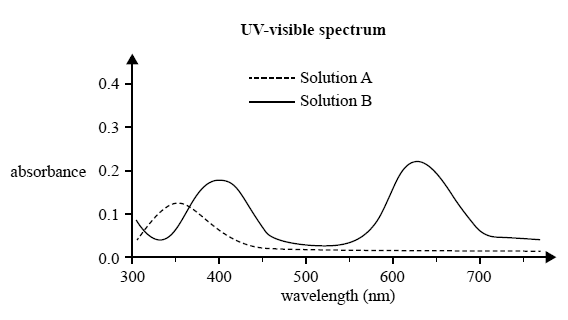a) If 10.00 mL of Solution A was mixed with 10.00 mL of Solution B, which wavelength should be used to measure the absorbance of Solution B in this mixture? Justify your answer. Solutionb) The analyst used two sets of standard solutions and blanks to determine the calibration curves for the two solutions. The absorbances were plotted on the same axes. The graph is shown below.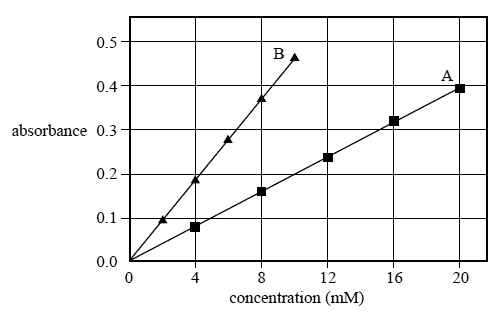The analyst found that, when it was measured at the appropriate wavelength, Solution A had an absorbance of 0.2 If Solution A was cobalt(II) nitrate, Co(NO3)2, determine its concentration in mg L–1. M(Co(NO3)2) = 182.9 g mol–1 1 mM = 10–3 M Solutionc) In another mixture, the pink compound in Solution A and the green compound in Solution B each have a concentration of approximately 1.5 × 10–2 M. Could the analyst reliably use both of the calibration curves to determine the concentrations for Solution A and Solution B by UV-visible spectroscopy? Justify your answer. Solution2) While cleaning out a laboratory shelf labelled 'Carboxylic acids and esters', a chemist discovers a bottle simply labelled 'C5H10O2'. To identify the molecular structure of the contents of the bottle, a sample is submitted for analysis using infrared spectroscopy, and 1H and 13C NMR spectroscopy. The spectra are shown below.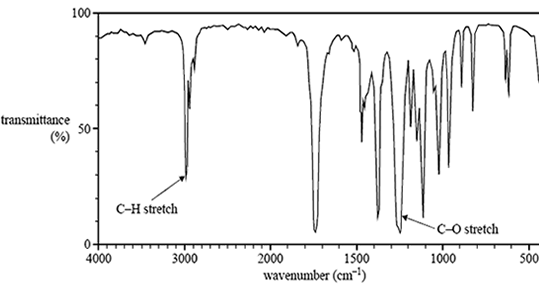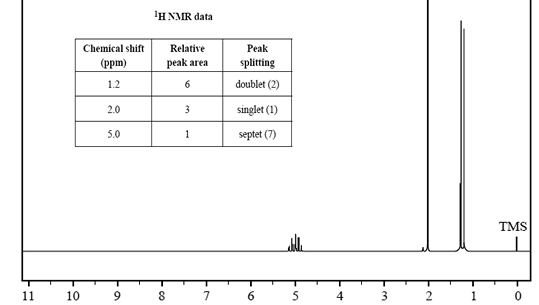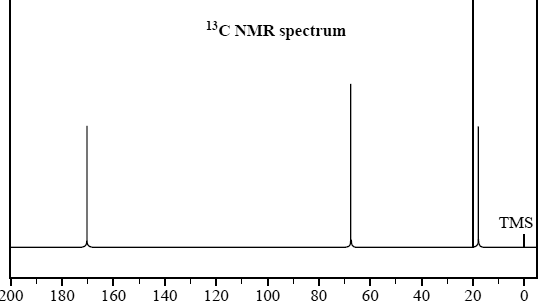a) Based on the IR spectrum, determine whether the molecule is a carboxylic acid or an ester. Provide a reason for your answer. Solutionb) Use the information provided in the 1H and 13C NMR spectra to identify the number of different chemical environments for hydrogen and carbon in this molecule.   Solutionc) Draw a structure for this molecule. Solution3) Which two isomers of C3H6Br2 have two peaks (other than the TMS peak) in their 13C NMR spectrum?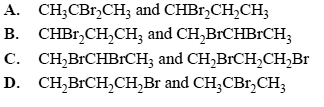Solution4) The high-resolution proton NMR spectrum of chloroethane has two sets of peaks. Both peaks are split. Which of the following correctly describes the splitting pattern? A. a singlet and a doublet B. a doublet and a doublet C. a doublet and a triplet D. a triplet and a quartet Solution5) Electromagnetic radiation of a specifi c wavelength can interact with some molecules and atoms by promoting electrons at a low energy level to higher energy levels.Which pair of analytical techniques relies on the measurement of these electronic transitions? A. atomic absorption spectroscopy and UV-visible spectroscopy B. infrared spectroscopy and atomic absorption spectroscopy C. proton NMR spectroscopy and UV-visible spectroscopy D. mass spectrometry and infrared spectroscopy Solution6) A small sample of a purified ester mixture was passed through a gas chromatograph (GC) attached to a mass spectrometer. The chromatogram showed two peaks, indicating that the ester mixture contained two different fatty acid methyl esters, A and B. The peak area of each compound and the mass-to-charge ratio of the molecular ion of each compound are shown in the following table. Assume that the charge on each molecular ion is +1.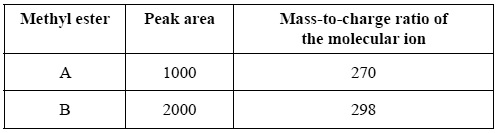The mass spectrum of methyl ester A corresponds to that of methyl palmitate, CH3(CH2)14COOCH3. What are the name and semi-structural formula of methyl ester B? (Refer to 'Formulas of some fatty acids' in the data book.) Solution